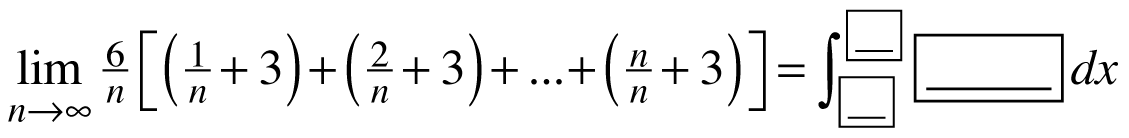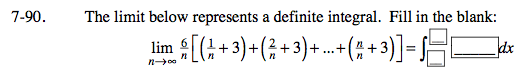### Home > CALC > Chapter 7 > Lesson 7.2.3 > Problem7-90

7-90.

The limit below represents a definite integral. Fill in the blank:
Homework Help ✎To make this easier, factor the 6 out of the limit.
The 6 will be factored out of the integral as well.
We will now write all n-terms as x-terms.

Find dx:

$\text{observe that:}\lim_{\Delta x\rightarrow 0}=\lim_{n\rightarrow 0}\frac{1}{n}.$

$\text{...so}\frac{1}{n}\text{ represents }\Delta x, \text{ and }\lim_{n\rightarrow \infty }\frac{1}{n}=dx$

Find the integrand:
x is a variable. So find the part of the sum that changes:

$\frac{1}{n},\frac{2}{n},\frac{3}{n},...\frac{n}{n}.$

So the integrand must be f(x) = x + 3.

$6\lim_{n\rightarrow \infty }\left [ \left ( \frac{1}{n}+3 \right )+ \left( \frac{2}{n}+3 \right )+...+\left( \frac{n}{n}+3 \right )\right ]=6\int_{a}^{b}f(x)dx$

$6\lim_{n\rightarrow \infty }\Delta x\left [ \left ( \frac{1}{n}+3 \right )+ \left( \frac{2}{n}+3 \right )+...+\left( \frac{n}{n}+3 \right )\right ]=6\int_{a}^{b}f(x)dx$

$6\lim_{n\rightarrow \infty }\Delta x(x+3)=6\int_{a}^{b}(x+3)dx { \ \ \ \ \ \ \ \ \ \ \ \ \ \ \ \ \ \ \ \ \ \ \ \ \ \ \ \ \ \ \ \ \ \ \ \ \ \ }$

$6\lim_{n\rightarrow \infty }\Delta x(x+3)=6\int_{0}^{1}(x+3)dx { \ \ \ \ \ \ \ \ \ \ \ \ \ \ \ \ \ \ \ \ \ \ \ \ \ \ \ \ \ \ \ \ \ \ \ \ \ \ }$

Find the bounds:
Look at the original sum.

$\text{The smallest value of }x \text{ was }\frac{1}{n},\text{ so }\lim_{n\rightarrow \infty }\frac{1}{n}=0, \text{ that's the lower bound.}$

$\text{The largest value of }x \text{ was }\frac{n}{n},\text{ so }\lim_{n\rightarrow \infty }\frac{n}{n}=1, \text{ that's the upper bound.}$